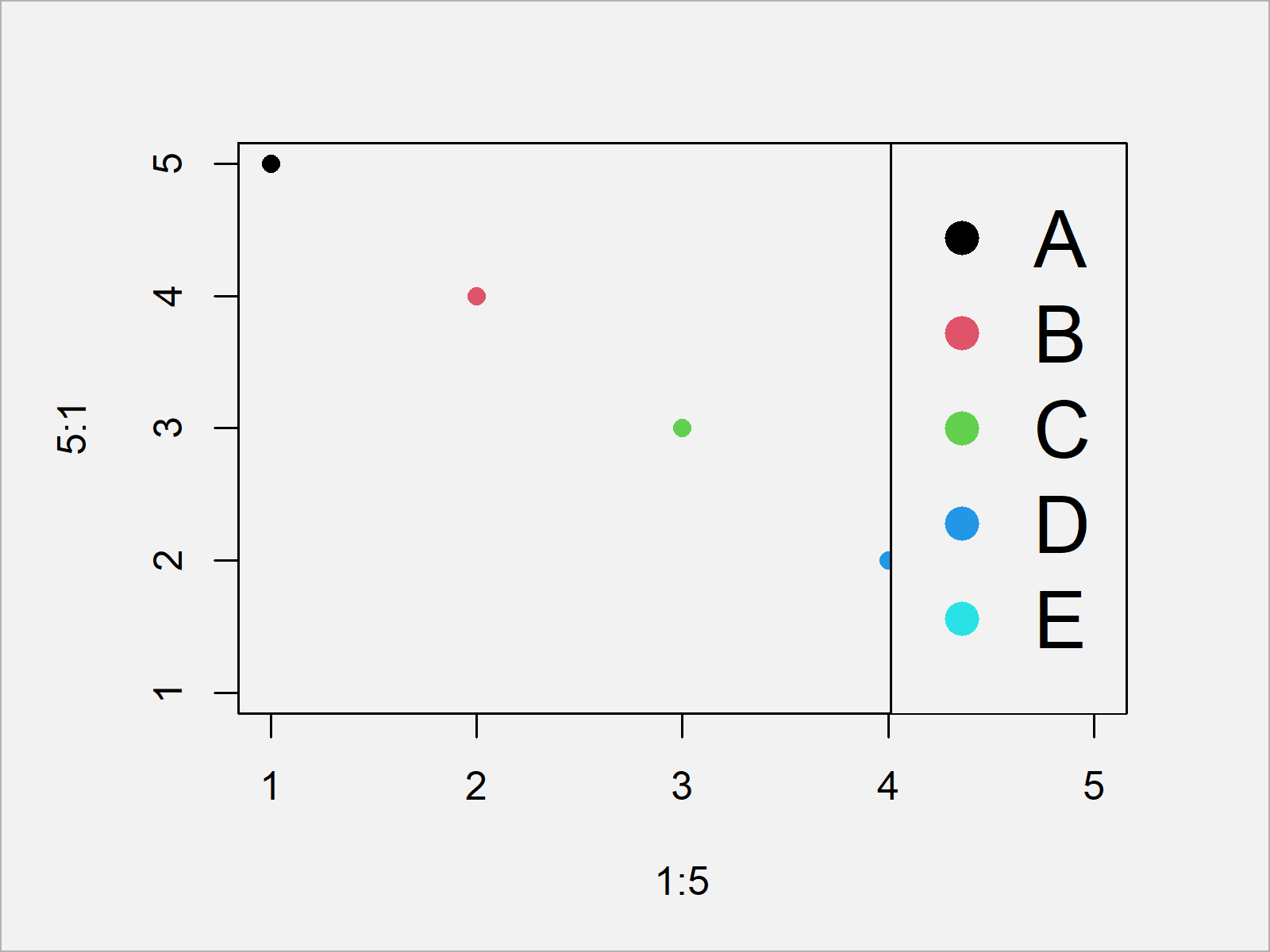# Change Font & Item Size in Base R Plot Legend (Example)

In this tutorial you’ll learn how to change font and item size in a plot legend in R.

Let’s take a look at some R codes in action:

## Draw Base R Plot with Default Legend

Let’s assume that we want to draw a Base R scatterplot with legend. Then, we could do that using the following R code:

```plot(1:5, 5:1,            # Create plot
col = 1:5,
pch = 16)
legend("topright",        # Create legend
legend = LETTERS[1:5],
pch = 16,
col = 1:5)```By running the previous syntax, we have plotted Figure 1, i.e. a Base R scatterplot with five legend items and item descriptions.

Now let’s assume that we want to adjust the font size of our legend text as well as of the corresponding legend items.

In the following example, I’ll explain how to do that!

## Example: Increase size of Base R Plot Legend Using par() Function

In this example, I’ll demonstrate how to increase the size of the font and the items in a Base R legend.

For this, we first have to draw our plot without legend:

```plot(1:5, 5:1,            # Create plot
col = 1:5,
pch = 16)```

In the next step, we have to modify the global options of the current R session. We can do that using the par function:

`op <- par(cex = 2)        # Change & save options`

Note that the previous code has also created a data object called op, which is containing the previously used options. More on that later.

Next, we can add the legend to our plot:

```legend("topright",        # Create legend
legend = LETTERS[1:5],
pch = 16,
col = 1:5)```As shown in Figure 2, we have plotted a legend on top of our plot that has much larger text elements and items. Looks good!

Finally, we have to restore the plot options of our R session:

`par(op)                   # Restore options options`

Note that this little trick helps to create a larger (or smaller) legend in a Base R graphic. In case you would like to increase or decrease a ggplot2 legend, you could have a look at this tutorial.

## Video & Further Resources

Do you need further info on the R programming code of the present tutorial? Then you could have a look at the following video on my YouTube channel. I’m explaining the contents of this tutorial in the video:

Also, you might have a look at the related articles on this website. A selection of articles is listed below:

In this R tutorial you have learned how to modify the font and item size in a plot legend. Please let me know in the comments, in case you have any further comments and/or questions.

Subscribe to the Statistics Globe Newsletter